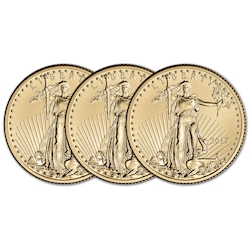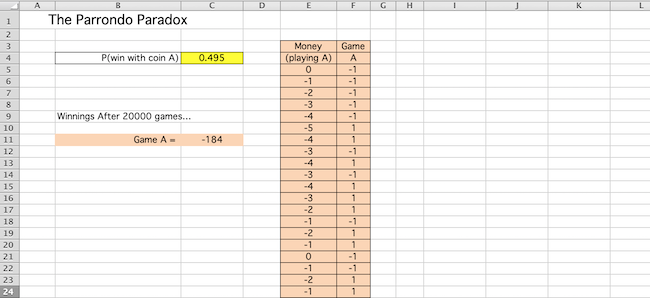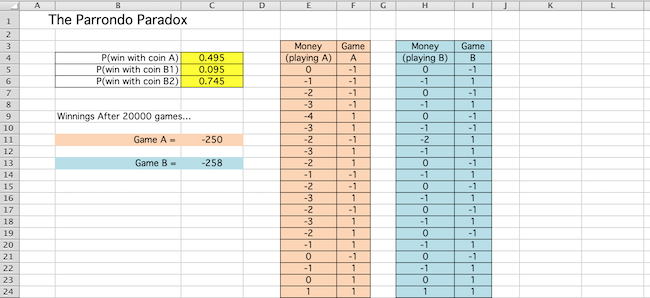The Parrondo Paradox has to do with taking two horrible situations and turning them into something beautiful. But let's not spoil the result before we even begin. Let's introduce two games instead:

Game A

This one couldn't be simpler. You have a single biased coin. When flipped, this coin only produces heads 49.5% of the time, and tails the rest of the time. You flip the coin. If it is heads, you win a dollar. If it is tails you lose a dollar. That's it -- like I said, it's as simple as they come.

Game B

This game requires two biased coins. One produces heads fairly rarely -- only 9.5% of the time. Let us call this coin B1. The other produces heads a much more frequent 74.5% of the time, on average (call it B2). To play this game, we will choose one of these coins and flip it. If we get heads, we again win a dollar -- and if we get tails, we again lose a dollar. We just need to know which coin to flip...

Suppose it's your birthday and your room-mate randomly picked some number of dollars (between 0 and 8, inclusive) and put them in your otherwise empty wallet, as a gift. If you counted up the dollar bills in your wallet and divided the total by 3, wouldn't you have an equal likelihood of getting a remainder of 0, 1, or 2? (Note, 0, 3, and 6 produce a remainder of 0; while 1, 4, and 7 produce a remainder of 1; and 2, 5, and 8 produce a remainder of 2.)

Let's use this as a means to choose the coin to flip in a way that involves some randomness. Every time you want to play game B:

1. count your money (in numbers of dollar bills);
2. divide that by 3 and find the remainder; and
3. if the remainder is 0 flip coin B1, while if the remainder is 1 or 2 then flip coin B2

Which of these two games would you rather play?

Certainly, the first game looks like a losing game on average, since one wins less than half the time.

Game B looks more promising. Indeed, you have just learned some techniques in your statistics class for calculating probabilities, and you decide to use them to help you find the probability of winning game B. Under the assumption that one-third of the time you flip coin B1 and two-thirds of the time you flip coin B2, and given the respective probabilities of winning with these coins of $0.095$ and $0.745$, you calculate the probability of winning game B as $$P(\textrm{Win Game B}) = (1/3)(0.095) + (2/3)(0.745) \doteq 0.5283$$

Not surprisingly, you decide playing game B not only is the better game, but it might eventually win you some money over the long haul. So you start playing game B. But after several rounds, you appear to have lost money. Is this just bad luck? ..or is something more curious going on?

To test whether your original analysis was correct, you decide to model both games in Excel -- simulating the cash won or lost after playing both games for a long time.

Let's start with the easier of the two games to simulate, Game A...

On a new Excel worksheet, fill out cells B4:C4, B1, B9, B11, and E3:F4 to agree with what is shown below, and set the amount of money initially in your wallet to some random value of your choosing between 0 and 8, inclusive, by putting this number in cell E5.Then, enter a formula in F5 that can be copied and pasted to cells below this cell, so that each cell "plays game A", returning either $1$ or $-1$ in accordance to whether the game was won or lost, respectively. Your formula should reference the probability seen in C4, allowing you to change the probability of winning game A throughout the worksheet by only changing the value in this one yellow cell.

Each cell below E5 should report the sum of the money won or lost from the last game played (i.e., the value in the previous row, column F) and however much money one had previously (i.e., the value in the previous row, same column). Enter an appropriate formula in E6 to this end.

We would like to get a good feeling for the potential long term impact on one's wallet, so let us play this game an obscene number of times. As such, copy the formulas in E6 and F5 and paste them into cells to fill a range long enough vertically to represent 20,000 games played. (The picture above only shows these (orange) cells down to row 24, but you get the idea.)

So that we don't have to scroll forever to see how much money you have after 20,000 games played, enter a formula in C11 to see this final value easily.

Hit the F9 key several times to simulate several trials of 20,000 games played. Are the results in C11 what you expected?

Now let's do something very similar to simulate game B.

Fill cells H3:I4, B5:C6, and B13 as shown below, and initialize your new "money" column by putting a random value between $0$ and $8$ inclusive, into cell H5.Create a formula in I5 that can be copied and pasted below it, so each resulting cell "plays game B", again returning either $1$ or $-1$ in accordance to whether the game was won or lost, respectively. Note that each such cell in column I will need to reference not only the cells C5 and C6 for the probabilities there, but also the cell representing the current amount of money one has (i.e., the cell to the immediate left), so that the right decision can be made with regard to which coin should be flipped.

Enter a formula in H6 to keep track of your total amount of money over time, just like was done in column E previously. Then, add a formula to cell C13 to show the final amount of money one has after 20,000 games of game B played.

Was the result what you expected?

You are not sure why, but looking at the evidence, it appears Game B is a losing game!

Befuddled as you are about why this might be, you realize that you might be able to use this unexpected result to your advantage to pay off some debt you owe to your math major room-mate -- a result of a rather large "trouncing" you took in a recent poker game.

You tell your room-mate about the two games, showing off your calculations for the predicted probabilities (but not revealing the results of your recent simulations). You acknowledge that it looks like, based on your calculations that game B wins slightly more often than 50% of the time (recall you got 52.83%), but you are feeling lucky, and would be willing to go "double-or-nothing" on that poker debt you owe him. If he just plays game B a quick 50 times, you explain, and ends up making money as a result of these games, you'll pay him twice what you owe him in addition to the money he just won. But -- if he loses money after his 50 games, the poker debt you owe him must be forgiven, and he needs to pay you whatever he just lost playing game B.

Your room-mate counters -- "What if I play both games A and B -- like if every third time I play game A, playing game B the other two times?" Knowing as you do that both games are losing games, how can you refuse? "Sure!" you reply -- secretly thinking your room-mate is a bigger sucker than you thought -- everybody can see that game A is a losing game!

(50 games later...)

"How is that possible?", you ask -- as you realize you now owe your room-mate even more money. ""I guess today just wasn't your lucky day, sorry.", he replies.

Feeling somehow that your math major room-mate may have just tricked you (you've got to watch out for math majors -- we are a sneaky lot!), you decide to simulate this third situation in Excel as well.

Set up columns K and L and cells B15:C15 to simulate the results of playing the sequence of "losing games" ABB, ABB, ABB,... (20,000 times), as suggested by what is below:As before, use F9 to simulate many trials of 20,000 games played. Typically, what happens? How can this be?

How can one have their money go up, while playing two games that are both working to make it go down?

Hmmm...